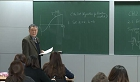### 주메뉴

### 수치해석(I)

• 울산대학교
• 김용인• 주제분류
자연과학 >수학ㆍ물리ㆍ천문ㆍ지리 >수학
• 강의학기
2012년 1학기
• 조회수
5,939
•
In this lecture, we focus on the numerical solutions of equations with one variavle. We begin with the elementary numerical methods such as bisection method and secant method, and then proceed to Newtons method, one point iteration method and Mullers method. Each method is followed by numerical experiments on the computer. In addition, in order for the students to be familiarized to using Mathematica, four Mathematica Quizs will be imposed to the students.
Course Outline Bisection method Numercal experimen#### 차시별 강의1.Course Outline Bisection method Numercal experimen2.Bisection method (1)Bisection method (2)3.False position method and Newtons method (1)False position method and Newtons method (2)4.Numercal experiments (1)Numercal experiments (2)5.Iteration mathod (1)Iteration mathod (2)6.Iteration mathod (3)Iteration mathod (4)7.Aitken extrapolation (1)Aitken extrapolation (2)8.Modified Newtons method (1)Modified Newtons method (2)9.Mullers method (1)Mullers method (2)10.Eulers method (1)Eulers method (2)#### 연관 자료#### 사용자 의견

강의 평가를 위해서는 로그인 해주세요.

#### 이용방법

• 강의 이용시 필요한 프로그램 [바로가기]

※ 강의별로 교수님의 사정에 따라 전체 차시 중 일부 차시만 공개되는 경우가 있으니 양해 부탁드립니다.